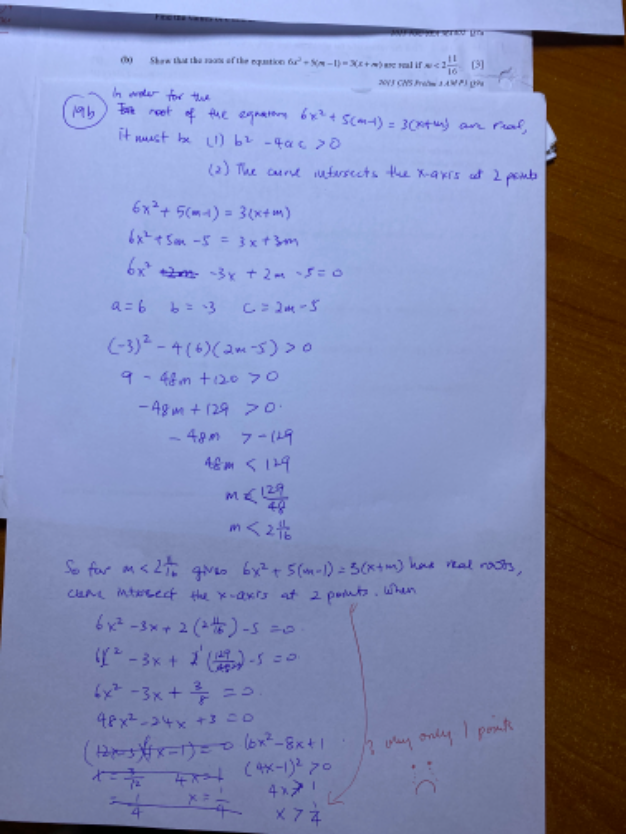# QuestionKindly show me how to present on this question, is really burden me down. Thanks

Your hypothesis one can be assumed as this to prove that m < 2 11/16.

Assume that the intersection of the two functions has real roots.

this means D > 0

6x2 -3x + 2m -5 = 0

a = 6, b = -3 , c =2m-5

(-3)2 – 4(6)(2m-5)  > 0

9 – 48m + 120 > 0

-48m  > -129

48m < 129

m < 129/48

m < 2  11/16

Hence 6x2 + 5(m-1) = 3(x + m) has real root if m < 2  11/16.

I also found one x = 1/4 (the same as you) and further D = 0 for the resulting quadratic, suggesting that the line is tangent at that one point on the curve.  So I am not able to find 2 roots as stated in your second hypothesis.